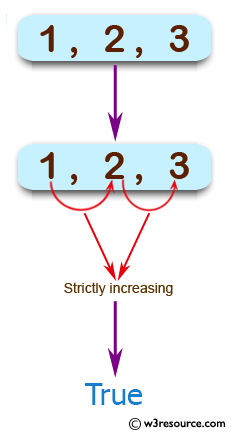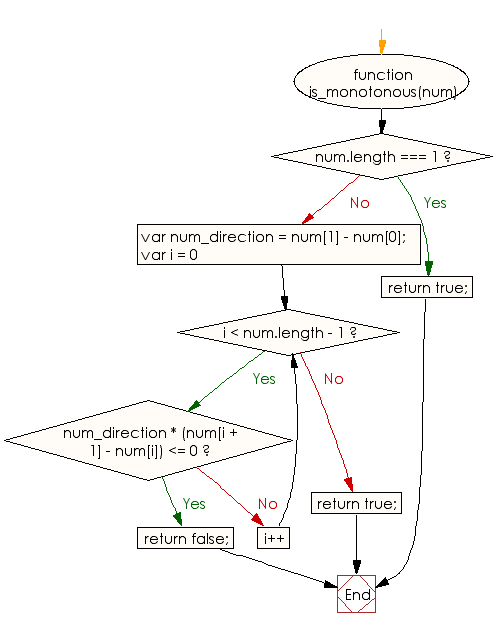# JavaScript: Check whether a given array of integers represents either a strictly increasing or a strictly decreasing sequence

## JavaScript Basic: Exercise-122 with Solution

Write a JavaScript program to check whether a given array of integers represents either a strictly increasing or a strictly decreasing sequence.

Pictorial Presentation:Sample Solution:

HTML Code:

``````<!DOCTYPE html>
<html>
<meta charset="utf-8">
<meta name="viewport" content="width=device-width">
<title> Check whether a given array of integers represents either a strictly increasing or a strictly decreasing sequence</title>
<body>

</body>
</html>
```
```

JavaScript Code:

``````function is_monotonous(num) {
if (num.length === 1) {
return true;
}
var num_direction = num - num;
for (var i = 0; i < num.length - 1; i++) {
if (num_direction * (num[i + 1] - num[i]) <= 0) {
return false;
}
}
return true;
}
console.log(is_monotonous([1, 2, 3]));
console.log(is_monotonous([1, 2, 2]))
console.log(is_monotonous([-3, -2, -1]))
``````

Sample Output:

```true
false
true
```

Flowchart:ES6 Version:

``````function is_monotonous(num) {
if (num.length === 1) {
return true;
}
const num_direction = num - num;
for (let i = 0; i < num.length - 1; i++) {
if (num_direction * (num[i + 1] - num[i]) <= 0) {
return false;
}
}
return true;
}
console.log(is_monotonous([1, 2, 3]));
console.log(is_monotonous([1, 2, 2]))
console.log(is_monotonous([-3, -2, -1]))
``````

Live Demo:

See the Pen javascript-basic-exercise-122 by w3resource (@w3resource) on CodePen.

Improve this sample solution and post your code through Disqus

What is the difficulty level of this exercise?

Test your Programming skills with w3resource's quiz.

﻿

## JavaScript: Tips of the Day

Returns the sum of the powers of all the numbers from start to end (both inclusive)

Example:

```const sumPower = (end, power = 2, start = 1) =>
Array(end + 1 - start)
.fill(0)
.map((x, i) => (i + start) ** power)
.reduce((a, b) => a + b, 0);
console.log(sumPower(10)); // 385
console.log(sumPower(10, 3)); // 3025
console.log(sumPower(10, 3, 5)); // 2925
```

Output:

```385
3025
2925
```